## Facebook Hacker Cup 2013: qualification round problem analysis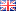As in previous years, I will be competing in the Facebook Hacker Cup, and I will describe the solutions I come up with on this weblog, hoping that other programmers or fellow competitors find them interesting.

I try to balance brevity with rigor: pasting just my solution code would not be very informative, but detailed proofs get boring quickly. Aiming for a happy medium, I will describe my solution approach before presenting the corresponding source code, adding proof outlines where necessary and linking to Wikipedia for detailed explanations of well-known topics.

This post contains source code written in Python. Unfortunately, Tweakers.net persists in their failure to support syntax highlighting for this popular language, which is why you will see screen shots below (but don't worry: links to the raw source code are provided as well).

#### Problem A: Beautiful Strings (20 points)

(Full problem statement here.)

We are asked to maximize the total “beauty” of a string, calculated as the sum of the beauty of the letters in the string, by assigning optimal values to different letters. The intuitive approach is to greedily assign the highest value (26) to the most common letter, the next highest value (25) to the next most common letter, and so on. Before coding this up, let's try to prove that the intuition is correct.

Formally, if we call value(x) the assigned value of letter x, and count(x) the number of times it occurs in the input string, then the total beauty equals the sum of count(x) × value(x) for all x, and we claim that a valuation is optimal if (and only if): value(x) > value(y) if count(x) > count(y).

This condition is necessary, because if value(x) > value(y) while count(x) < count(y), then swapping the values would increase the total beauty by (value(x) - value(y)) × (count(y) - count(x)) and therefore such a valuation cannot be optimal. The condition is also sufficient, because exchanging values for letters which occur equally often does not change the total beauty.

Now that we have proven the greedy approach to be correct, we can implement it in Python as follows: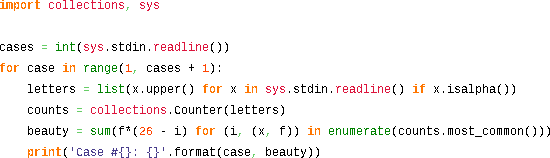#### Problem B: Balanced Smileys (35 points)

(Full problem statement here.)

If we ignore the smileys for a moment, the problem reduces to checking if all parentheses in the input are properly balanced. We can check this in linear time by scanning the string once (e.g. from left to right) and tracking the current nesting depth, which is increased for every opening parenthesis we encounter, and decreased for every closing parenthesis.

Using this approach, the string is well-formed if and only if:
1. we end at nesting depth 0, and
2. the nesting depth never drops below 0.
For example, this is a string with balanced parentheses:
 Input text: Nesting depth: a ( b ( c ) d ( e ) ) f ( g ) h 0 0 1 1 2 2 1 1 2 2 1 0 0 1 1 0 0

But this string has an unmatched opening parenthesis, and thus violates rule 1:
 Input text: Nesting depth: a ( b ( c ) d 0 0 1 1 2 2 1 1

And this string has an unmatched closing parethesis, which violates rule 2:
 Input text: Nesting depth: a ) b ( c 0 0 -1 -1 0 0

This approach works well with just parentheses, but the presence of smileys complicates matters, because we don't know in advance if we should count them as parentheses or not. Fortunately, we can adapt the above algorithm to deal with this uncertainty. Instead of tracking a single nesting depth value at each position, we should keep track of a set of integers representing all possible nesting depths.

Since this set will necessarily consist of consecutive integers, we can just store the minimum and maximum elements (knowing that all values in between are possible too). Again, we conclude that the string is well-formed if the lower-bound at the end is 0, and the upper bound never becomes negative (which would imply the set of possibilities is empty).

For example, this string is well-formed:

 Input text: Lower bound: Upper bound: ( ( : ) : ) ) 0 1 2 1 0 0 0 1 2 2 2 1

This idea can be implemented succinctly in Python: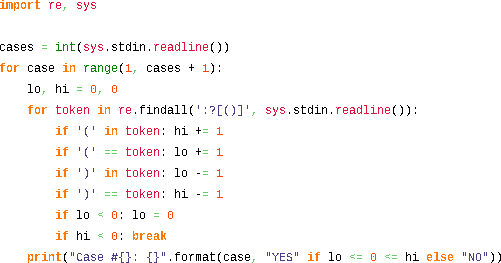Note that this solution asymptotically optimal: it requires linear time and constant space.

#### Problem C: Find The Min (45 points)

(Full problem statement here.)

The final problem looks complicated, with all the parameters and formulas described in the problem statement, but we can approach it systematically by breaking it down into simpler subproblems.

First, the problem statement dictates that the input is generated using a pseudo-random linear congruential generator. This is only done to keep the size of the input files small, so we can generate the first K elements of the array using the the provided formula, and then forget about the RNG parameters for the rest of the problem.

Although these first K values could be anything, we can make some useful observations about the contents of the array after the initial K elements:
1. Every element will be between 0 and K (inclusive) by the pigeonhole principle.
2. Consequently, every window of K + 1 consecutive elements will contain each value between 0 and K exactly once (i.e. it contains a permutation of the integers 0 through K).
3. Consequently, for i > 2K: M[i] = M[i - (K + 1)].
The final conclusion is useful because it implies that the generated array is cyclic with period K + 1. Below is a simple example with K = 4, N = 18, where this is property is clear:
 Index Value 0 1 2 3 4 5 6 7 8 9 10 11 12 13 14 15 16 17 3 1 4 1 0 2 3 4 1 0 2 3 4 1 0 2 3 4

This means that if we can compute the elements at indices K through 2K (inclusive), we have effectively computed them all. K is not ridiculously large (at most 100,000) but we should still be somewhat efficient in our implementation. I used a sliding window algorithm in which the array is calculated from left to right, while two data structures are maintained that contain information about the preceding K elements which is used to quickly calculated new elements.

The first data structure counts how often each distinct value is present in the window of K preceding elements. This could be a simple array of K+1 integers (though I found Python's Counter class slightly more convenient).

The second data structure is an ordered collection of integers (between 0 and K, inclusive) that are missing in the same window. Of course, I want to take the minimum element from this list at each step, and I want to be able to update it efficiently. Therefore, a plain list isn't the right choice. Instead, I will use a heap structure, although an ordered binary search tree (like Java's TreeSet or C++'s std::set) would also be appropriate.

Note that the present and missing data structures complement each other: if a value is stored in missing, then its count in present will be zero. And vice versa: if a value is not in missing then it must appear in the current window, and its count in present will be nonzero

Now consider how these data structures are updated when the window slides to the right. First, to determine M[i] for an index i ≥ K, I can remove the lowest value from the missing set, and then increment present\[M[i]], thus extending the window on the right by one element. To shrink the window on the left, I need to decrement present\[M[i - K]]. If the resulting count has reached zero, that means M[i - K] doesn't occur anywhere else in the search window, and it should be added to missing.

The implementation in Python looks like this: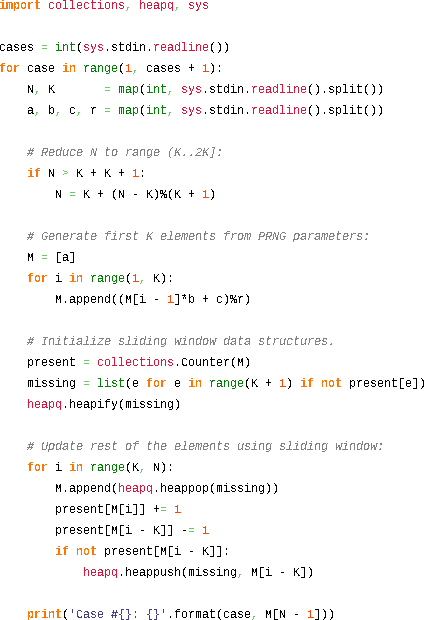Since heap operations on a list of size O(K) take O(log K) time, this algorithm runs in O(K×log K) time and O(K) space. Although this is fast enough for this contest, I suspect this is not optimal, and O(K) time should be possible too. If you know how to do it, please leave a comment describing your approach!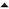02-'13 Facebook Hacker Cup 2013: round 1 problem analysis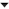06-'12 Using netcat to build a simple TCP proxy in Linux

I got another solution for number 3, it worked on all the test cases and ran the official input file in 1.5 second using ruby (macbook 2008 core 2 duo. 0.5 seconds on my i5 desktop)

I found out that you can generate the recurring list of K-2K items backwards without really using a sliding window. I called it the 'k_row' in my code. Also I ofcourse only generated enough items backwards until the index was reached that gave the correct answer.

Basically I found out that you can generate a normal 0..K range and then compare each item backwardly with the M item at the same index. If the M item is smaller, it needs to be inserted at that position and deleted from the initial range. Then you move to the next position to check that one. However, you need to check that you don't delete a number more than one time, therefor the hashset to check if an item already has been deleted once.

I can't really explain why it works, it was more the result of experimentation.

code:
 ```1 2 3 4 5 6 7 8 9 10 11 12 13 14 15 16 17 18 19 20 21 22 23 24 25 26 27 28 29 30 31 32 33 34 35 36 37 38 39 40 41 42 43 44 45 46 47 48 49 50 51 52 ``` ```def find_min(a,b,c,r,k,n) #Generate initial numbers with the given generator m = [] m << a (k - 1).times do |k| m << ((b * m[k] + c) % r) end last_k_items = m[-k..-1] #Calculate what the negative index is (backwards index from end) for the required item on position n. item_index = (n % k) - (n/k) negative_index = item_index < 0 item_index = item_index.abs % last_k_items.size item_index = 0 - item_index if negative_index if (!negative_index) item_index = item_index - (k+1) end #Generate the cyclic row of K items k_row = generate_k_row(last_k_items, k, item_index) #RESULT return k_row[item_index] end def generate_k_row(m,k,negative_index) #Initial row. Just 0,1,2,3...K k_row = (0..k).to_a deleted = Set.new #Traverse the k_row from end until required index is in the list. start = k-1 ending = k + negative_index #Very important: otherwise you use 0 and generate all the items. (much!) (start.downto ending).each do |i| m_i = m[i] if (m_i > k_row[i+1]) #Do nothing else if (!deleted.include?(m_i)) k_row.delete(m_i) deleted << m_i k_row.insert(i+1,m_i) end end end return k_row end```

[Comment edited on dinsdag 29 januari 2013 16:13]

I am a bit confused about problem B though.

I must admit first that I am not able to run your python script at the moment and that I am far from experienced in it. But If I interpret your explanation and the code correctly the string "I am super happy (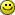) " will lead to a NO, while it is balanced, right?
Or am I misinterpreting your code/algorithm?

For problem 3, I originally did the same approach you did. Eventually I realized that while generating the first k elements in the PRNG, any time I encountered a number that fell between 0 and k, I could store a mapping of that value to the highest index it appeared at in the first k elements. Using your example above, the mapping would include { 1 : 3 }.

What this mapping means for us when it comes time to generate the second set of k elements starting at m[k], is that (e.g.) 1 will stil be in our rolling window until we reach m[k+3+1]. When we have a mapping for any in-range numbers that happened to appear in the first k elements, we can just start filling in a "recurrence array" (an array of size k+1 where we store the repeating sequence). We can do it optimistically, mean inning just start by trying to put 0 at recurrence_array. If 0 is in our mapping though, we know it won't be available for our recurrence array until mapping+1.

Since we start filling with the value 0, if we find it in the mapping we can be sure that as soon as 0 becomes first available we're going to want to use it (since there is nothing smaller). So the upshot is that we can safely just put 0 at recurrence_array[mapping+1].

At this point, the algorithm falls out of the set up. You just loop from 0..k+1, trying to stuff the value into the next available slot unless the value appears in the mapping, in which case you must place it at mapping[value]+1. A slot is "available" if a smaller number hasn't already been stuffed into it (i.e. it is null).

So in all, you only loop from 0..k twice an store only a mapping structure and an array of size k+1

I used Dynamic Programming for problem 2, but this is a much more elegant solution!
For the other problems, my solution was about the same. Other than that I used C++ instead of Python.

@Tommy: that's an excellent approach, and a good explanation!

@-peter-: I think your program implements the same idea, but in reverse for some reason.

I think it's clever that you basically inverted the problem: instead of generating the elements M[k] through M[2k] in order, you place the values 0 through K at their appropriate locations in M. I hadn't thought of this, but clearly this was the key to a linear-time solution. Thanks for posting!

[Comment edited on dinsdag 29 januari 2013 16:36]

Someone please explain for which test case my code fails for the problem#1

#include<stdio.h>
#include<algorithm>
#include<iostream>
#include<string>
using namespace std;
int main()
{
int t,i,l,j,temp,k;
string a,chumma;
int n;
cin>>t;
getline(cin,chumma);

for(i=1;i<=t;i++)
{
l=0;
for(k=0;k<26;k++)
n[k]=0;
getline(cin,a);
printf("Case #%d: ",i);

for(j=0;a[j]!='\0';j++)
{
if(a[j]>=65&&a[j]<=90){
n[a[j]-65]++;
}
else if(a[j]>=97&&a[j]<=122){
n[a[j]-97]++;
}
// cout<<a;
}
sort(n,n+26);
//for(i=0;i<26;i++)
//printf("%d ",n[i]);
for(k=0;k<26;k++)
{
temp=n[k]*(k+1);
//printf("%d ",n[k]);
l+=temp;
}
if(i<t)
printf("%d\n",l);
else
printf("%d",l);
}
return 0;
}

Balabarath: I don't know. The only possible problem I see is that you omitted the newline character on the last line of output; you don't need to do that, but I wouldn't expect you to fail because of that either. Maybe you just uploaded the wrong file?

Your explanation of Example B is puzzling me.
Shouldn't the Lower bound end with Input text: ( ( : ) : ) ) be -1?
In which case the string is well-formed if the lower-bound at the end is less than or equal to 0, and the upper bound never becomes negative.
That's also how I interpret the last line: print("case #{}: {}".format(case, "YES" if lo <= 0 <= hi else "NO"))

[Comment edited on donderdag 31 januari 2013 14:22]

@Vaan Banaan:

I think I've confused you by talking about sets of possibilities first, and then using two variables (lo/hi) to describe that set. The set of possible depths should never contain negative values (because negative depths aren't allowed anywhere); a negative value for hi just indicates an empty set.

Perhaps the idea behind the algorithm is more clear if I use a set explicitly:

Python:
 ```1 2 3 4 5 6 7 8 9 10 11 ``` ```depths = set() for token in re.findall(':?[()]', sys.stdin.readline()): if '(' in token: new_depths = set(depth + 1 for depth in depths) if ')' in token: new_depths = set(depth - 1 for depth in depths if depth > 0) if ':' in token: depths = depths.union(new_depths) else: depths = new_depths print("Case #{}: {}".format(case, "YES" if 0 in depths else "NO"))```

Note that here “depths” contains an explicit set of possible nesting depths, and we answer “YES” if and only if 0 is in the final set of depths. The code I originally posted (using hi/lo variables) is just an optimized version of the same idea.

[Comment edited on vrijdag 1 februari 2013 17:17]

Although the qualification round was over last week and my submission to Q3 failed (because of my script's performance, I still spent some time to attempt to optimize my code. After some examination I realize that the problem could be possibly solved within seconds (which I guess is just the same solution as the Ruby script above)

After some analysis it is understandable that the array will repeat itself at some point and all we need to generate for the series is actually the 0th element up to 2k-th (therefore 2k + 1 elements) unless n is smaller than 2k+1.

The first k elements must be generated with the LCG. Then, the (k+1)-th element must be generated by doing a tally on the occurrence of the first k elements (as some numbers may repeat). A dict called remaining_numbers which consists of k elements of 0s is created. (this dict needs only to be of k+1 size only because the elements after k-th element is minimum number of previous k items and therefore it is not possible that it would be bigger than k+1.)

After doing this tally, we walk through the dict sequentially from the beginning, finding out the first item that has 0 count, then the index indicates the minimum number that has never appeared in previous k items. Thus we don't care if the number is the first k elements is > k in value. Now we should then got the k-th (0-indexed) item in the list. Besides, we should keep this tally to the tally dict (named remaining_numbers) created too.

The (k+1)th to (2k)-th elements can be found by making use of the tally list created above. We can reduce the number of operations by just -1 count for the k+1 item earlier than the current element. At this point, we may go through the tally dict (remaining_numbers) to find the first item that has 0 count. But we can again cut the search operation again by checking if the (k+1) item earlier than the current item is smaller than the 1 item earlier than the current item. If so, the minimum should actually be this (k+1) earlier item, if not, we should search for the minimum from the 1 item earlier than the current item by checking its count. This should cut the amount of searches a lot and perhaps achieve O(n) complexity.

Pardon me for my poor explanation ... anyways please find the code below:

#!/usr/bin/python

line_number = 0
#f = 'sample_input.txt'
f = 'find_the_mintxt.txt'

for line in open (f, 'r'):
if line_number == 0:
#this is the number of test case
test_case_count = int(line)
elif (line_number/2) <= test_case_count:
if (line_number%2 == 1):
(n,k) = line.split(' ')
n = int(n)
k = int(k)
else: #if line_number%2 == 0
#print "Case #"+str(line_number)+": "+str(compute_string(line.strip()))
(a,b,c,r) = line.split(' ')
a = int(a)
b = int(b)
c = int(c)
r = int(r)

b = b % r
m = {}
#print (n,k,a,b,c,r)
#try to generate the sequence out

#for i in range(0, n): #whole series has n items
i = 0
m = a

for i in range(1,k):
m[i] = (b * m[i-1]%r + c) % r

#at this moment, i == k
remaining_numbers =  * (k + 1)
for j in range(k):
if (m[j] < k):
remaining_numbers[m[j]] += 1
for pindex, p in enumerate(remaining_numbers):
if p == 0:
m[k] = pindex
break
remaining_numbers[m[k]] += 1

for i in range(k+1, min((2*k+1), n)):
j = i - k - 1
if (m[j] < k):
remaining_numbers[m[j]] -= 1

if ((m[j] < m[i-1]) and (remaining_numbers[m[j]] == 0)):
m[i] = m[j]
remaining_numbers[m[j]] += 1
else:
j = m[i-1] + 1
while (remaining_numbers[j] > 0):
j += 1
m[i] = j
remaining_numbers[j] += 1

if (n > (2*k+1)):
ans = m[(n-k)%(k+1) + k - 1]
else:
ans = m.values().pop()
print "Case #"+str(line_number/2)+": "+str(ans)
line_number += 1

sorry the formatting messed up... please see my code in pastebin:
http://pastebin.com/muBHGsCN